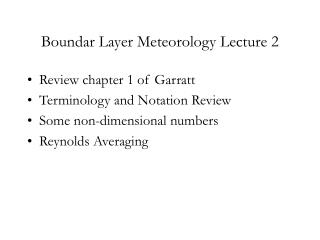DownloadDownload PresentationBoundar Layer Meteorology Lecture 2

# Boundar Layer Meteorology Lecture 2

Télécharger la présentation## Boundar Layer Meteorology Lecture 2

- - - - - - - - - - - - - - - - - - - - - - - - - - - E N D - - - - - - - - - - - - - - - - - - - - - - - - - - -
##### Presentation Transcript

1. Boundar Layer Meteorology Lecture 2 • Review chapter 1 of Garratt • Terminology and Notation Review • Some non-dimensional numbers • Reynolds Averaging

2. Review chapter 1 of Garratt • Inner and outer layers (what’s with this, is the outer layer really part of the boundary layer?) • Seasonal and geographic variations of the boundary layer’s character.

3. Modeled Boundary Layer Depth

4. Modeled Boundary Layer Depth

5. Observed Boundary Layer Depth

6. Terminology • Boundary Layer Regions: • Surface Layer • Mixed Layer • Residual Layer • Stable (Nocturnal Boundary) Layer • Entrainment Zone • Ekman Layer (Outer Layer) • Surface Layer

7. Boundary Layer Regions

8. Notation • Variables: T, Tv ,, v , , q, x, y, z, • Viscosity:  = du/dy; Tkg/(m s)=Pa/s • Kinetmatic viscosity: m2/s • Summation (Einstein) notation: see Stull handout (pp. 57-74). Note definitions of the Kronecker delta (and distinction between it and the unit vector), and the alternating unit tensor (Levi-Civita symbol) used to express the cross product.

9. Some Non-Dimensional Numbers • Reynolds number:Re = VL/ • Reynolds number is ratio of acceleration (or “inertial force”) to friction force. It governs transition to turbulence (at high Reynolds numbers , e.g. about 2300 for pipes; highly variable!). • Richardson numbers: ratio of • Flux • Gradient: Ri = (g/ddz)/(du/dz)2 • Bulk

10. Reynolds averaging and Reynolds Stresses t1 should be enough larger than t2 so that the average is independent of time.

11. Reynolds averaging and Reynolds Stresses

12. Understanding Reynolds Stress